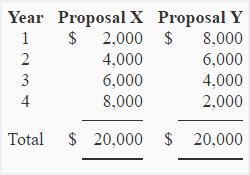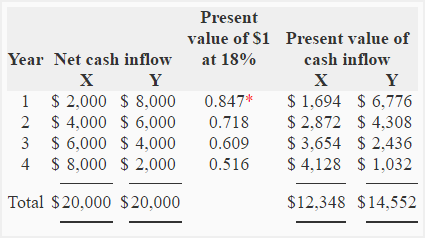# Exercise-4 (Net present value method – uneven cash flows)

Proposal X and proposal Y require an initial investment of \$10,000 and are expected to generate an equal cash inflow of \$20,000 over their life of four years. The net cash inflow for each year of life of both the proposals is given below:Required:

1. Compute the present value of cash inflows generated by both the proposals assuming a discount rate of 18%.
2. Which of the two proposals is better if compared using net present value method?

## Solution:

(1). Present values of proposal X and proposal Y:

Both the proposals generate uneven cash inflows. The present value of cash inflow of both the proposals would, therefore, be computed by multiplying the net cash inflow generated in each individual year by the present value of \$1 at given interest rate of 18%. These calculations are given below:*Value from present value of \$1 table.

Notice that both the investment proposals generate equal cash inflow of \$20,000 in 4-year period but present value of proposal Y’s cash inflow is greater than the present value of proposal X’s cash inflow by \$2,204 . The reason is that proposal Y generates most of its cash inflow in earlier years whereas proposal X generates most of its cash inflow in later years. It reminds us that the money has a time value.

(2). Comparison of two proposals using net present value (NPV) method:

NPV = Initial cost – Present value of cash inflows

• NPV of proposal X: \$10,000 – \$12,348 = \$2,348
• NPV of proposal Y: \$10,000 – \$14,552 = \$4,552

The net present value of both the proposals are positive and therefore both the proposals are acceptable if evaluated using net present value method. The proposal Y, however, promises a higher net present value than proposal X and is therefore a better investment to choose.

A D V E R T I S E M E N T
3 Comments on Exercise-4 (Net present value method – uneven cash flows)
1.Nasir

It is very useful presentation but I need more examples.

2.Francesca

All you did was give the present value of the cash flows, not the net present value, which should take into consideration the initial 40,000 invested. Both these investment projects have a negative NPV, since the cash flows barely cover the initial cost.

1.Accounting For Management

The exercise was missing its second part in which the NPV of both the proposals was computed and compared.